Circle - simple

The circumference of a circle is 930 mm. How long in mm is its diameter?

Result

D =  296.03 mm

Solution:Leave us a comment of example and its solution (i.e. if it is still somewhat unclear...):Be the first to comment!Next similar examples:

1. Bicycle wheel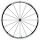Bicycle wheel diameter is 62 cm. How many times turns the bicycle on the road 1 km long?
2. Clock handsThe second hand has a length of 1.5 cm. How long does the endpoint of this hand travel in one day?
3. Athlete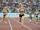How long length run athlete when the track is circular shape of radius 120 meters and an athlete runs five times in the circuit?
4. Circle from string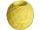Martin has a long 628 mm string . He makes circle from it. Calculate the radius of the circle.
5. WellRope with a bucket is fixed on the shaft with the wheel. The shaft has a diameter 50 cm. How many meters will drop bucket when the wheels turn 15 times?
6. Velocipede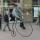The front wheel of velocipede from year 1880 had a diameter 1.8 m. If the front wheel turned again one then rear wheel 6 times. What was the diameter of the rear wheel?
7. Odometer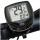The odometer is driven by rotation of the wheel whose diameter is 65 cm. After how many rotations the wheel turns counter to next kilometer?
8. Circle r,DCalculate the diameter and radius of the circle if it has length 52.45 cm.
9. MineWheel in traction tower has a diameter 5 m. How many meters will perform an elevator cabin if wheel rotates in the same direction 49 times?
10. FlowerbedIn the park there is a large circular flowerbed with a diameter of 12 m. Jakub circulated him ten times and the smaller Vojtoseven times. How many meters each went by and how many meters did Jakub run more than Vojta?
11. CircleWhat is the radius of the circle whose perimeter is 6 cm?
12. Bicycle wheelAfter driving 157 m bicycle wheel rotates 100 times. What is the radius of the wheel in cm?
13. Circle - easy 2The circle has a radius 6 cm. Calculate:
14. 22/7 circleCalculate approximately area of a circle with radius 20 cm. When calculating π use 22/7.
15. Two circlesTwo circles with a radius 4 cm and 3 cm have a center distance 0.5cm. How many common points have these circles?
16. AnnulusThe radius of the larger circle is 8cm, the radius of smaller is 5cm. Calculate the contents of the annulus.
17. Pizza 4Marcus ate half pizza on monday night. He than ate one third of the remaining pizza on Tuesday. Which of the following expressions show how much pizza marcus ate in total?